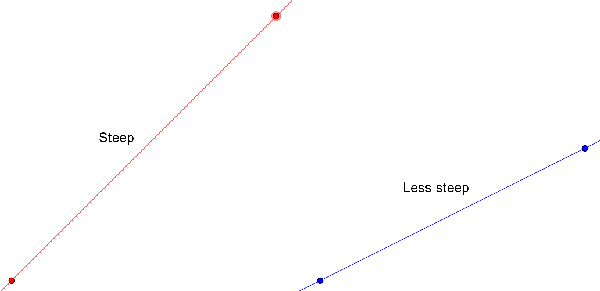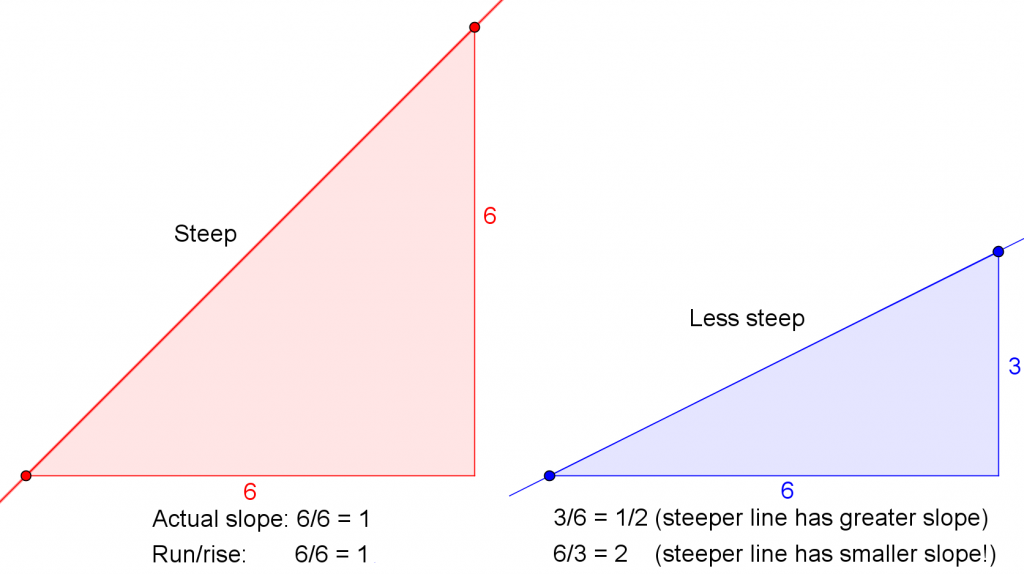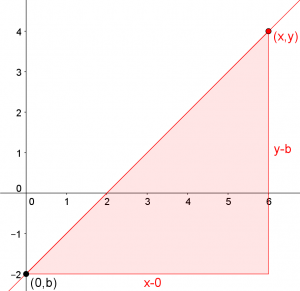# Why Isn’t Slope Run Over Rise?

Definitions are interesting in several ways. Sometimes they are essentially arbitrary; other times there is a very good reason for them, and understanding that reason can be helpful in understanding and using them. But they are usually taught just as something to memorize. Let’s think about why slope is defined as it is, and not some other way. I’ll look at three answers we’ve given, all saying similar things, but from somewhat different perspectives.

## Right for the word, right for the equation

Here is a 2005 question:

Why Is Slope Rise Over Run and Not Run Over Rise?

Someone asked our math teacher yesterday, why is it rise over run and not run over rise... I was wondering since our teacher didn't know the answer, if you might.  If you could answer this that would be so great!!!

I think that maybe it could be both ways because like for instance: (I'm going to show my work here)

(4,2)(3,-7)
2-(-7) / 4-3 = 9/1
y=mx+b
1/9 + 2
y=9/1x + 2

and when you graph it you put the dot at the 2 place and go up 9 lines and go out one line and put a dot (then connect those dots) BUT you can also put a dot at the 2 go out one, and up 9 and put a dot there (then connect) and it's the same thing.  Do you think rise over run can also be run over rise???

Kristin’s thoughts focus on how you draw a line with a given slope: you can either go up 9 and then right 1, or right 1 and then up 9. But these are both a slope of 9/1 = 9; they are just two ways to get to the same point. Kristin didn’t relate these to the equation, to see whether both equations give the same point.

But I saw two separate points to discuss:

There are at least two questions here: why do we define the word "slope" to mean the ratio of "rise" to "run", and why is that the right number to use in the slope-intercept form of a line?

The answer to the first question is that any number we assign to a "slope" ought to be bigger when the line is sloped more steeply. We want the "slope" to tell us how much the line is sloped. A steeper line goes up more in the same distance:If we used the "run" over the "rise", then the less steep line would have a greater slope, which wouldn't make sense:So the ratio as we define it fits the word “slope”, by being greater when the line slopes more. Now the second issue:

How about the question of what goes in the "m" spot in the equation? Let's look at the intercept and one other point on the line:What is the slope of that line?

y-b   y-b
m = --- = ---
x-0    x

If we multiply both sides of the equation by x, we get

mx = y - b

and adding b to both sides gives

mx + b = y

So if we define slope as rise/run, then this is the equation that any point on the line has to fit. If you defined slope differently, you would get a different equation.

So here's how it works: we define slope in a way that makes sense based on what the word "slope" means; then we find that we can use that slope value in an equation that describes any point on the line. One could use a different formula for something like "slope", and get a different equation; but this one gives us a reasonable definition AND a nice little equation to use it in.

I didn’t follow up on the idea of getting a different equation using the upside-down definition. Let’s do that now. Suppose we define “new slope” as $$\displaystyle n = \frac{x_2-x_1}{y_2-y_1}$$. Then, in the situation above, we have $$\displaystyle n = \frac{x}{y-b}$$, leading to the equation $$\displaystyle y = \frac{x}{n} + b$$. Nothing really wrong with that as an equation … but then we would have students asking why we have to divide by n rather than multiplying by n. And that would motivate flipping the definition of n.

## Capturing the idea of steepness

Doctor Ian gave similar reasoning here, in 2008:

Why Does the Slope Formula Work?

To find the slope of a line, you do the formula y2-y1/x2-x1, when x1 is not equal to x2.  But why does this formula work?  I don't understand the relationship of the subtraction and division with slope.  Is it simply an equation that creates different numbers which signify different angles?

His focus was on gaining an understanding by going through the process of inventing the concept oneself:

In many cases, the best way to understand something is to re-invent it for yourself.

Suppose I have two lines, like

B                       C
.                    .
.                .
.          .
.     .
A

We would say that AB is "steeper" than AC, right?  But how could you QUANTIFY that?  What kind of calculation could you do to attach a number to the idea of "steepness"?

That's what we're doing with slope.  We're trying to capture that concept in a calculation.

Now, in the case of AB and AC, they both have the same amount of vertical travel ("rise").  But AB has less horizontal travel ("run"). If we divide rise by run, we get a larger number for AB, which is nice, because it makes sense that a larger value should correspond to a steeper line.

Now, where does subtraction come in?  Well, suppose we think of each line segment as the hypotenuse of a right triangle:

B                       C
.  |                 .     |
.     |          .            |
.        | .                     |
.     .     |                       |
A______________|_______________________|

If we know the coordinates of A, B, and C, can you see how we can use subtraction to find the rise and run for each segment?

Another point might be worth making here: Wouldn’t we get an equally good measure of steepness (maybe even more natural) by making it rise/drive — that is the rise divided by the distance we actually travel along the line? On the surface, that makes sense; the only problem is that the equations you’d get would be horrendous. The slope formula would become $$\displaystyle s = \frac{y_2 – y_1}{\sqrt{(x_2 – x_1)^2 + (y_2 – y_1)^2}}$$, and the equation of the line would be $$\displaystyle y = \frac{sx}{\sqrt{1 – s^2}} + b$$, which amounts to converting our new slope s to the familiar m before using it.

My conclusion: It is really the convenience of the equation, more than anything, that drives the choice of definition.

## A name for what the coefficient does

By 2012 I had changed my own perspective to what I just stated, so I was glad of an opportunity to answer the question again.

Here is the question that came in then, from teacher Sherrie, who gave just the right setup for what I wanted to say:

Why Y Rises and X Runs

I have researched online for a reason why slope is y over x, and have not found any answers.

The formula can be derived by the point-slope form of a line, but which came first -- the chicken or the egg?

I have usually told my class that some guy (because apparently it was the guys who used to do all the math) arbitrarily decided that we were going to look at the change of a line as rise over run rather than the other way around.

I started with the usual reasoning based on the meaning of the word “slope”:

We'd like "slope" to be a number that is greater when a line is more sloped (steeper), and smaller (by which I mean, closer to zero) when the line is less sloped.

So the slope should say how fast the line RISES as you move forward. That makes it the ratio of the distance risen to the distance moved forward, just as speed (how fast you move forward as time passes) is measured as the ratio of distance moved to time elapsed.

One way to answer this kind of question (even if you don't know the answer ahead of time) is to experiment with alternatives. Draw a few lines (say, with slopes 1/2, 1, and 2) and note what they look like; then calculate what their "slopes" would be if you defined them as change in x over change in y. Which numbers make more sense as a measure of slope?

Then I dealt with the equation in a new way, starting with the equation:

You're also right that the concept of slope can be derived from the equation of a line. Starting with the simplest form, y = ax + b, you can ask what "a" and "b" MEAN. You find that "b" is the point on the y-axis where the line crosses (so you invent the term "y-intercept" and decide that it is useful). You also find that "a" represents the rate at which the line rises. (By the way, in America we traditionally call that "m" for no good reason; "a" just tends to get used in other ways). This leads you to decide that this ratio is also useful, and the name "slope" makes good sense for it.

So, the name "slope" fits the definition "change in y over change in x," and that concept is useful because of slope-intercept form. Maybe the egg came first, but the chicken made it worth incubating!

From this perspective, it is quite possible that the simple form of the equation came first, with no particular names attached to the coefficients; and the names were given based on their meaning. So maybe someone didn’t come along and ask, “How shall we define something called ‘slope’?”, but rather, they recognized that this concept fit well with a basic linear equation, so it deserved a name – and “slope” fit perfectly!

Incidentally, this can also explain why the names we give the parameters (m and b in the U.S., various other combinations elsewhere) don’t stand for anything (like “s” for “slope”, as many students ask us). They are just parameters in the equation, which happen to have meanings, rather than starting out as something with a meaning (like “r” for radius in the equation of a circle). For more on this, see

Why b for Intercept?

You may also find this relevant:

Order in Linear Expressions

I had a little more to add:

One more thought: As students move on, they will find slope showing up in many other places, where the same definition is still useful. It represents the rate of change of y with respect to x, and therefore the rate at which any function changes. In calculus, this becomes the derivative. In physics, it is a speed. In business, it may show up as a price per unit, or as a cost per hour. A slope is a rate, and it is always the ratio of the change in the dependent variable to the change in the independent variable. So a third reason we define slope as we do is that it is a very fertile concept that becomes more and more important as you learn more.

And that is often the ultimate reason for a definition: it becomes popular because it makes subsequent math work well. All the ideas I listed are just different names for the same concept, and all must be defined as “output change over input change” in order both to mean what we want, and to behave conveniently (for example, you can add speeds together).

This reminds me of a case where both orders are used. In the U.S., we measure the efficiency of a car in miles per gallon (mpg): how many miles you get per gallon used. In some metric countries, kilometers per liter (km/L) is used similarly. Here a larger number is better; this ratio represents efficiency, and we are thinking of fuel as input and miles driven as output. (That makes sense: It’s what a car does!)

But in other places, the usual measurement is liters per 100 kilometers (L/100 km). This number represents the rate of usage: how much fuel you use to go a certain distance. Here a smaller number is better. They are thinking of distance driven as input and fuel usage as the result. (That makes sense, too: It’s what you do when you drive a car.)

Both approaches, though opposite, make sense; the first is most natural as part of figuring out how far you can go with the fuel you have, while the second is most natural for deciding how much fuel it will take to go a certain distance.

But always, a rate is the same as a slope: the rate of change of an output per unit change of input. What varies is what we are thinking of as the output.

### 4 thoughts on “Why Isn’t Slope Run Over Rise?”

1.Very very helpful, please send any link to follow you are your ideas. This page helped me a lot Dave!

1.I’m glad the page is helpful!

Thanks for calling my attention to the fact that the Subscribe option had disappeared. I have made it available now.

2.Thank you. I guess to sum it up we use rise/run because we measure the ratio of a line based off the slope’s incline intensity.

3.first thank you to create this page that help people
i am also want to add my logic that in every ratio the order is important and when we want to know the ratio of something to other the thing we want to know its ratio must be come in numerator so slope is also a kind of ratio and we cannot write rise in denominator because that change our slope name
thank you

This site uses Akismet to reduce spam. Learn how your comment data is processed.